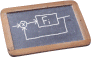Numerical Optimization

COURSE DESCRIPTION

Abstract

Optimization plays a key role in solving a large variety of decision problems that arise in engineering (design, process operations, embedded systems), data science, machine learning, business analytics, finance, economics, and many others. This course focuses on formulating optimization models and on the most popular numerical methods to solve them.

Syllabus

Modeling: linear programming models, convex optimization models. Basic optimization theory: optimality conditions, sensitivity, duality. Algorithms for constrained convex optimization: active-set methods for linear and quadratic programming, proximal methods and ADMM, stochastic gradient, interior-point methods. Line-search methods for unconstrained nonlinear programming, sequential quadratic programming.

PREREQUISITES

Linear algebra and matrix computation, calculus and mathematical analysis.

LOCATION

IMT School for Advanced Studies Lucca, Piazza San Francesco 19, Lucca, Italy

LECTURE SLIDES
• Optimization models. Linear and convex programming.
• Optimization theory (optimality conditions, duality)
• Basics of numerical linear algebra
• Active-set methods